# APPENDIX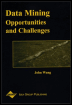Chapter XII - Mining Free Text for Structure Data Mining: Opportunities and Challenges by John Wang (ed) Idea Group Publishing 2003Brought to you by Team-Fly

Spreading activation is used to account for lexical variation between the clients' questions and the FAQ answers. Spreading activation is based on WordNet, which consists of four subnets organized by the four parts of speech. Each subnet has its own relations: for example, nouns have antonymy, the isa relation, and three part-of relations. WordNet's basic unit is a synset, which contains words and phrases interchangeable in a context, e.g., "computer" and "data processor."

The activation procedure is depth-constrained. It takes a term and a depth integer specifying how many links away from the term the activation is to spread. Each term found during the spread is annotated with its part of speech and the depth at which it was found. Thus, "device12" means that "device" is a noun found at depth 2. The origin term's depth is 0. If a word is found at several depths, only the smallest one is kept. Activation is spread only from terms found in the questions. Since questions in FAQs are shorter than answers, the number of non-relevant terms found during the spread is much smaller that it would be if the activation was spread from every nonstoplisted term in every answer.

The weight of a term combines its semantic and statistical properties. The semantic properties of a term constitute its intrinsic value. The statistical properties reflect its value in the collection of textual units. The semantic weight of a term ti, Wwn(ti,r), is given by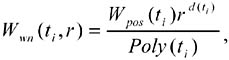where Poly(ti) gives the term's polysemy, d(ti) gives the depth at which ti was found, Wpos assigns a constant weight to each part of speech, i.e., 1 to nouns, .75 to verbs, and .5 to adjectives and adverbs, and the rate of decay, r < 1, indicates how much ti's weight decreases with depth.

The statistical weight of a term combines several approaches. Let K be a collection of D documents. Let dj be a document in K. If f(ti ,dj) denotes the frequency of occurrence of ti in dj, then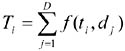denotes the number of occurrences of ti in K. Let Ni be the number of documents containing at least one occurrence of ti. Ni depends on the distribution of ti among the documents of K. Let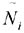be the random variable that assumes the values of Ni and let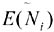be its expected value, assuming that each occurrence of ti can fall into any of the D documents with equal probability.

The first statistical weight metric is the inverse document frequency (Salton & McGill, 1983). The inverse document frequency (IDF) of ti in K, Widf ( ti , K ), is given by 1 + log(D/Ni). The tfidf weight of ti in dj, Wtfidf ( ti , dj ), is given by f ( ti , dj ) Widf ( ti , K ).

The second statistical weight metric is condensation clustering (Kulyukin, 1998b). A sequence of textual units proceeds from topic to topic. Terms pertinent to a topic exhibit a non-random tendency to condense in the units that cover the topic. One refers to such terms as content-bearing. Terms that do not bear content appear to be distributed randomly over the units. The condensation clustering (CC) weight of ti, Wcc ( ti , K ), is a ratio of the actual number of documents containing at least one occurrence of ti over the expected number of such documents and is given by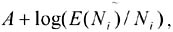, where A is a constant.

The following lemma shows how to compute the expectation of Ni.

Lemma 1: Let Ti be the total number of occurrences of ti in K. Then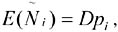, where pi = 1 (1 1/D)Ti.

Proof: For each dj, put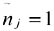if f(ti, dj) >0 and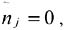, otherwise. This random variable assumes the values of 1 and 0 with corresponding probabilities of pi and 1pi. Hence,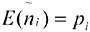. Since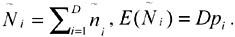.

The CC weight of ti captures its importance in K. To account for ti's importance in dj, its CC weight is multiplied by its frequency in dj. Thus, we obtain another statistical weight metric Wtfcc (ti, dj, K) = f (ti , dj) Wcc (ti , K). The following lemma captures the relationship between IDF and CC.

Lemma 2: Wcc (ti) = Widf (ti) + log (pi)

Proof: By lemma 1 and the definition of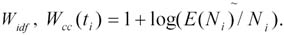. But,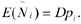. Hence, Wcc (ti) = Widf (ti) + log (pi), for A = 1.

 Brought to you by Team-Fly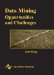Data Mining: Opportunities and Challenges
ISBN: 1591400511
EAN: 2147483647
Year: 2003
Pages: 194
Authors: John Wang